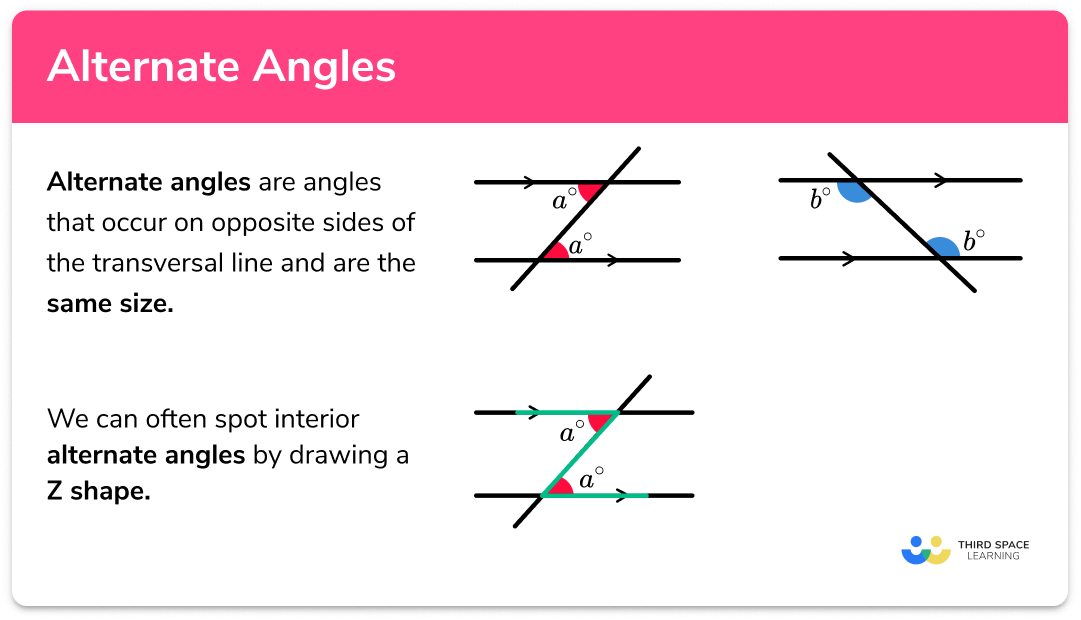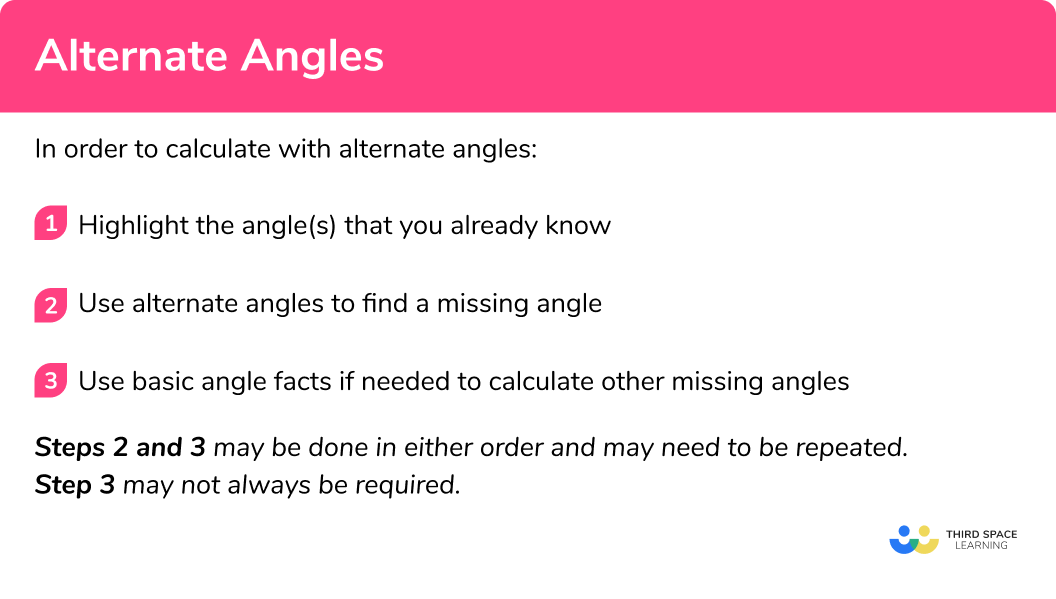# Alternate Angles

Here we will learn about alternate angles including how to recognise when angles are alternate and apply this understanding to solve problems.

There are also angles in parallel lines worksheets based on Edexcel, AQA and OCR exam questions, along with further guidance on where to go next if you’re still stuck.

## What are alternate angles?

Alternate angles are angles that occur on opposite sides of the transversal line and have the same size

Alternate angles are equal:

We can often spot interior alternate angles by drawing a Z shape:

There are two different types of alternate angles, alternate interior angles and alternate exterior angles

Below are diagrams showing these two variations.

Alternate interior angles:

Here, the two angles of a and b are in between the parallel lines and therefore are pairs of alternate interior angles.

Alternate exterior angles:

Here, the two angles of c and d are outside of the parallel lines and so these are two examples of pairs of alternate exterior angles.

It is important to notice that the transversal on each diagram is at a different angle but the two angles in each diagram are the same size. As is the case with all diagrams for angles in parallel lines, never use a protractor to find an angle as all the diagrams, unless stated otherwise, are not to scale.

### What are alternate angles?### How to calculate with alternate angles

In order to calculate with alternate angles:

1. Highlight the angle(s) that you already know.
2. Use alternate angles to find a missing angle.
3. Use a basic angle fact to calculate the missing angle.

### How to calculate with alternate angles.## Alternate angles examples

### Example 1: alternate angles

1. Highlight the angle(s) that you already know.

2State the alternate angle, co-interior angle or corresponding angle fact to find a missing angle in the diagram.

Here we can label the alternate angle on the diagram as 50°.

3Use a basic angle fact to calculate the missing angle.

Here as θ is on a straight line with 50°.

\begin{aligned} \theta&=180^{\circ}-50^{\circ} \\ \theta&=130^{\circ} \end{aligned}

### Example 2:  alternate angles

Here we can state the angle 62° as it is corresponding to the original angle.

The angle θ is the alternate angle to the sum of 56° and 62° as shown in the diagram below.

\begin{aligned} \theta&=56^{\circ}+62^{\circ} \\ \theta&=118^{\circ} \end{aligned}

### Example 3: alternate angles with algebra

By working out the value for x, calculate the size of the missing angle θ. Justify your answer.

Here we know most of the angles so highlight the angles that are going to help you find the value of x.

Here, 3x and 30° are alternate to each other. We can therefore find the value for x:

\begin{aligned} 3x&=30^{\circ} \\ x&=10^{\circ} \end{aligned}

Here we can use the sum of angles in a triangle to help us calculate the value for θ. The value for 10x=100° as x=10°. We therefore can calculate θ:

\begin{aligned} \theta&=180^{\circ}-(100^{\circ}+30^{\circ}) \\ \theta&=50^{\circ} \end{aligned}

### Common misconceptions

• Mixing up angle facts

There are a lot of angle facts and it is easy to mistake alternate angles with corresponding angles. To prevent this from occurring, think about the alternate angles being on the alternate sides of the line.

• Using a protractor to measure an angle.

Using a protractor to measure an angle. Most diagrams are not to scale and so using a protractor will not result in a correct answer unless it is a coincidence.

Alternate angles is part of our series of lessons to support revision on angles in parallel lines. You may find it helpful to start with the main angles in parallel lines lesson for a summary of what to expect, or use the step by step guides below for further detail on individual topics. Other lessons in this series include:

### Practice alternate angles questions

1. Calculate the size of angle \theta .\theta=76^{\circ}\theta=256^{\circ}\theta=84^{\circ}\theta=104^{\circ}104^{\circ} and \theta are alternate angles so \theta = 104^{\circ}.2. Calculate the size of angle \theta.\theta=103^{\circ}\theta=38.5^{\circ}\theta=77^{\circ}\theta=13^{\circ}Using alternate angles and angles on a straight line: \theta=180-103=77^{\circ}3. Find the value of \theta\theta=63^{\circ}\theta=117^{\circ}\theta=79^{\circ}\theta=101^{\circ}Using alternate angles, we can see that the angle in the bottom right vertex of the triangle is 38^{\circ} . We can then use angles in a triangle: \theta = 180-(79+38)=63^{\circ}4. Work of the value of \theta\theta=22^{\circ}\theta=158^{\circ}\theta=79^{\circ}\theta=68^{\circ}Using alternate angles, we can see the angle \theta=22^{\circ} . We can then use the fact that this is an isosceles triangle and the other two angles in the triangle are the same.

180-22=158
\begin{aligned} 158 \div 2 &= 79^{\circ}\\ \theta &= 79^{\circ} \end{aligned}5. Calculate the size of angle \theta\theta=110^{\circ}\theta=68^{\circ}\theta=70^{\circ}\theta=40^{\circ}Using alternate angles, we can see the angle 110^{\circ} . Then \theta=110-42=68^{\circ}6. By calculating the value for x , work out the value of each angle labelled.22^{\circ} \text{ and } 34^{\circ}34^{\circ} \text{ and } 34^{\circ}50^{\circ} \text{ and }130^{\circ}50^{\circ} \text{ and } 50^{\circ}Alternate angles are equal so 7x-20=4x+10When x=10 :
7x-20=710-20=50^{\circ}
4x+10=410+10=50^{\circ}

### Alternate Angles GCSE questions

1. Find the size of angle a . Give reasons for your answer.(3 marks)

180 – 70 = 110 since angles in a triangle add up to 180^{\circ}.

(1)

Isosceles triangle so two angles equal 110 \div 2 = 55.

(1)

Alternate angles are equal so a=55^{\circ}.

(1)

2. Find the size of angle y .(3 marks)

Angle ACD =72^{\circ}

(1)

Angle ACB = 180 - \left (90+48 \right ) = 42^{\circ}

(1)

##### 72-42=30^{\circ}

(1)

3. Show that the lines AB and CD are parallel.(4 marks)

(1)

(1)

##### \begin{aligned} 5 \times 21+3&=108\\ 6 \times 21 - 18&=108 \end{aligned}

(1)

Alternate angles are equal therefore the lines are parallel.

(1)

## Learning checklist

You have now learned how to:

• Apply the properties of angles at a point, angles at a point on a straight line, vertically opposite angles
• Understand and use the relationship between parallel lines and alternate and corresponding angles

## Still stuck?

Prepare your KS4 students for maths GCSEs success with Third Space Learning. Weekly online one to one GCSE maths revision lessons delivered by expert maths tutors.

Find out more about our GCSE maths tuition programme.